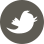# ACM暑期培训-51Nod-1383

8月 1, 2018 - 算法7=1+1+1+1+1+1+1
=1+1+1+1+1+2
=1+1+1+2+2
=1+2+2+2
=1+1+1+4
=1+2+4

Input

Output

Sample Input
7 Sample Output
6